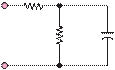### Create an Account

Already have account?

### Forgot Your Password ?

Home / Questions / Repeat Problem 61 for the circuit of Figure P62 500 Ù Figure P65 66 In ...

# Repeat Problem 61 for the circuit of Figure P62 500 Ù Figure P65 66 In the circuit shown in Figure P66 where C 05 μF and R 2 k2 a Determine how the input

Repeat Problem 6.1 for the circuit of Figure P6.2.

500 Ù

Figure P6.5

6.6     In the circuit shown in Figure P6.6, where

C  = 0.μF and = 2 k2,

a.    Determine how the input impedance

 (  ω) =

Z  j          Vjω) behaves at extremely high and low

Ijω)+

vin(t)

500 Ù

200 mF

+

vout(t)

frequencies.

b.    Find an expression for the impedance.

c.

 ωRC

Show that this expression can be manipulated into the form Zjω) 1 + 1 .

Jun 18 2020 View more View Less

#### Answer (Solved)Subscribe To Get Solution